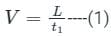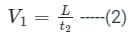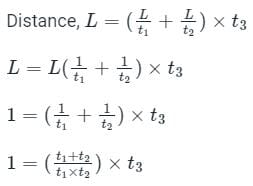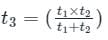ACT  >  Test: Relative Velocity in Two Dimensions

# Test: Relative Velocity in Two Dimensions

Test Description

## 10 Questions MCQ Test Physics for ACT | Test: Relative Velocity in Two Dimensions

Test: Relative Velocity in Two Dimensions for ACT 2023 is part of Physics for ACT preparation. The Test: Relative Velocity in Two Dimensions questions and answers have been prepared according to the ACT exam syllabus.The Test: Relative Velocity in Two Dimensions MCQs are made for ACT 2023 Exam. Find important definitions, questions, notes, meanings, examples, exercises, MCQs and online tests for Test: Relative Velocity in Two Dimensions below.
Solutions of Test: Relative Velocity in Two Dimensions questions in English are available as part of our Physics for ACT for ACT & Test: Relative Velocity in Two Dimensions solutions in Hindi for Physics for ACT course. Download more important topics, notes, lectures and mock test series for ACT Exam by signing up for free. Attempt Test: Relative Velocity in Two Dimensions | 10 questions in 15 minutes | Mock test for ACT preparation | Free important questions MCQ to study Physics for ACT for ACT Exam | Download free PDF with solutions
 1 Crore+ students have signed up on EduRev. Have you?
Test: Relative Velocity in Two Dimensions - Question 1

### A body is moving with a constant acceleration of 11î + 2ĵ, starting from î + ĵ. What will be the position of the body after 10s?

Detailed Solution for Test: Relative Velocity in Two Dimensions - Question 1

The body’s displacement is given by s = (1/2)at2. Therefore, after putting in the values, we get, s = (1/2)(11î + 2ĵ)*102 = 550î + 100ĵ. The body initially starts from î + ĵ. Hence, the final position is = initial position + displacement = 551î + 101ĵ.

Test: Relative Velocity in Two Dimensions - Question 2

### A body is moving with a constant velocity of 4î + 3ĵ, starting from the origin. What will be the position of the body after 10s?

Detailed Solution for Test: Relative Velocity in Two Dimensions - Question 2

The body’s displacement is given by s = vt. The body starts from the origin hence, the total displacement is the final position of the body. Therefore, after putting in the values, we get, s = (4î + 3ĵ)*10 = 40î + 30ĵ.

Test: Relative Velocity in Two Dimensions - Question 3

### Velocity of a man is 25 units in the positive X direction and 75 units in negative Y direction starting from the origin. Velocity of a woman is 25 units in the positive Y direction and 75 units in the negative X direction starting from the origin. What is the relative velocity of the man with respect to the woman?

Detailed Solution for Test: Relative Velocity in Two Dimensions - Question 3

The velocity of the man is 25î – 75ĵ and that of the woman is -75î + 25ĵ. Relative velocity = velocity of man – velocity of woman = 100î – 100ĵ.

Test: Relative Velocity in Two Dimensions - Question 4

The velocity of a car A is to 5î + 11ĵ. The velocity of another car B is 11î + 5ĵ. What is the relative velocity of B with respect to A?

Detailed Solution for Test: Relative Velocity in Two Dimensions - Question 4

The formula for calculating relative velocity of a body with respect to another body is- relative velocity = Velocity vector of A – Velocity vector of B. Hence, here, the relative velocity = velocity of B – velocity of A = 6î – 6ĵ.

Test: Relative Velocity in Two Dimensions - Question 5

A body is moving with a constant acceleration of 4î + 3ĵ, starting from the origin. What will be the position of the body after 5s?

Detailed Solution for Test: Relative Velocity in Two Dimensions - Question 5

The body’s displacement is given by s = (1/2)at2. The body starts from the origin hence, the total displacement is the final position of the body. Therefore, after putting in the values, we get, s = (1/2)(4î + 3ĵ)*52 = 50î + 37.5ĵ.

Test: Relative Velocity in Two Dimensions - Question 6

Velocity of a dog is 15 units in the positive X direction and 3 units in positive Y direction starting from the origin. Velocity of a pig is 7 units in the positive Y direction and 17 units in the positive X direction starting from the origin. What is the relative velocity of the dog with respect to the pig?

Detailed Solution for Test: Relative Velocity in Two Dimensions - Question 6

The velocity of the man is 15î+3ĵ and that of the woman is 17î + 7ĵ. Relative velocity = velocity of dog – velocity of pig = -2î – 4ĵ.

Test: Relative Velocity in Two Dimensions - Question 7

The velocity of a car A is to 5î. The velocity of another car B is 22î – 7ĵ. What is the relative velocity of A with respect to B?

Detailed Solution for Test: Relative Velocity in Two Dimensions - Question 7

The formula for calculating relative velocity of a body with respect to another body is- relative velocity = Velocity vector of A – Velocity vector of B. Hence, here, the relative velocity = velocity of B – velocity of A = -17î + 7ĵ.

Test: Relative Velocity in Two Dimensions - Question 8

When two bodies move uniformly towards each other, the distance decrease by 6 m/s. If both the bodies moves (as above) in the same direction with the same speed, the distance between them increases by 4 m/s. Then the speed of the two bodies are

Detailed Solution for Test: Relative Velocity in Two Dimensions - Question 8

Concept:

A) When two bodies move uniformly in opposite direction, the velocities gets added up.

B) When two bodies move uniformly in same direction, the velocities gets subtracted.

Calculation:

Given:

Let one bodies be A and B, and theor speed be V and U respectively.

As per the problem;

VA + VB = 6  ------(i)

VA - VB = 4  ------(ii)

Adding eq (i) and eq (ii)

2VA = 10

∴ VA = 5 m/s

Similarly

VA + VB = 6

5 + VB = 6

∴ VB  = 1 m/s

Test: Relative Velocity in Two Dimensions - Question 9

At a metro station, a girl walks up a stationary escalator in time t1 . If she remains stationary on the escalator, then the escalator take her up in time t2. The time taken by her to walk up on the moving escalator will be

Detailed Solution for Test: Relative Velocity in Two Dimensions - Question 9

In the situation of a stationary escalator and moving girl velocity,In the situation of stationary girl and moving escalator velocity,If both escalator and girl keep moving then distance,
L = (V + V1) t3   ----(3)
Where t3 is time to cover that distance when both girl and the escalator keep moving.
Using equations (1), (2), and (3) we get:Then, time is takenTest: Relative Velocity in Two Dimensions - Question 10

If  S = 3t- 5t + 7 find initial velocity.

Detailed Solution for Test: Relative Velocity in Two Dimensions - Question 10

Formula Used:
Velocity = s/t
Here, S is displacement and t is time
For instantaneous velocity, it expressed as dS/dt
Application:
We have,
S = 3t2 - 5t + 7
Hence,
Instantaneous velocity = dS/dt = (6t - 5)
initial velocity will be found at t = o
Hence,
V|initial = (6 × 0 - 5) = - 5

## Physics for ACT

41 videos|13 docs|16 tests
 Use Code STAYHOME200 and get INR 200 additional OFF Use Coupon Code
Information about Test: Relative Velocity in Two Dimensions Page
In this test you can find the Exam questions for Test: Relative Velocity in Two Dimensions solved & explained in the simplest way possible. Besides giving Questions and answers for Test: Relative Velocity in Two Dimensions, EduRev gives you an ample number of Online tests for practice

## Physics for ACT

41 videos|13 docs|16 tests